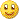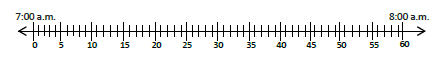3rd Module 2--Place Value and Problem Solving with Units of MeasureNew or Recently Introduced Terms

Analog Clock   a clock that has numbers around the outer edge with a minute hand and hour hand.4 + 4 = 8

capacity   the amount of liquid a container can hold.

Compose   change 10 smaller units into one larger unit

Decompose   break down of units

Gram   (g); unit of measurement for weight

Kilogram   (kg); unit of measurement for weightVolume   the space a liquid fills up (can be measure by L or mL)

Liter (L)
unit of measure for liquid volume (~1000mL)

Milliliter (mL)  unit of measure for liquid volumeVertical Number line
a number line that goes up and down (is vertical)Time as a number line   showing time using a number line

Topic A